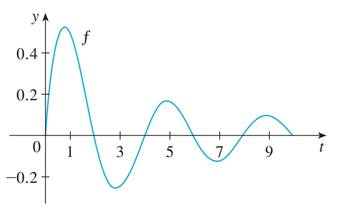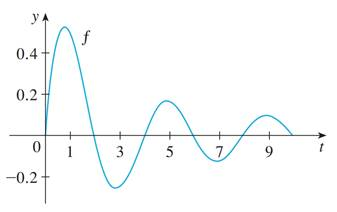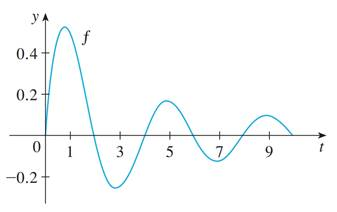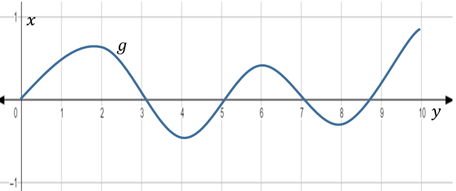# the value of x at which the local maximum and minimum values of g occur.### Single Variable Calculus: Concepts...

4th Edition
James Stewart
Publisher: Cengage Learning
ISBN: 9781337687805### Single Variable Calculus: Concepts...

4th Edition
James Stewart
Publisher: Cengage Learning
ISBN: 9781337687805

#### Solutions

Chapter 5.4, Problem 20E

a.

To determine

## To find: the value of x at which the local maximum and minimum values of g occur.

Expert Solution

The value of x at which the local maximum and minimum values of g occur at 2, 6 and 10 and at 4 and 8 respectively.

### Explanation of Solution

Given information:

The function is g(x)=0xf(t)dt and the graph of f(t) is shown below:Concept used:

The fundamental theorem of calculus, part 1 is defined by,

If f is continuous on [a,b] , then the function g defined by,

g(x)=axf(t)dt axb is an antiderivative of f , that is g(x)=f(x) for a<x<b .

Using Part 1 of the Fundamental Theorem of Calculus,

g(x)=f(x) [since, f(t) is continuous and 0<x<b ]

Since, a function has a local maximum or local minimum at a point x0 if and only if the first derivative of a function is equal to zero at x=x0 .

Hence, the function g has a local maximum and minimum at a point where g(x) that is f(x) is concave up and concave down respectively and touches the x -axis.

From the graph provided in the question, it can be observed that f is concave up between 0x2 , 4x6 and 8x10 and is concave down between 2x4 and 6x8 .

Therefore,

The value of x at which the local maximum and minimum values of g occur at 2, 6 and 10 and at 4 and 8 respectively.

b.

To determine

### To find: the value of x at which the absolute maximum values of g occur.

Expert Solution

The value of x at which the absolute maximum values of g occur at x=2 .

### Explanation of Solution

Given information:

The function is g(x)=0xf(t)dt and the graph of f(t) is shown below:Concept used:

The fundamental theorem of calculus, part 1 is defined by,

If f is continuous on [a,b] , then the function g defined by,

g(x)=axf(t)dt axb is an antiderivative of f , that is g(x)=f(x) for a<x<b .

Using Part 1 of the Fundamental Theorem of Calculus,

g(x)=f(x) [since, f(t) is continuous and 0<x<b ]

Since, a function has a local maximum or local minimum at a point x0 if and only if the first derivative of a function is equal to zero at x=x0 .

Hence, the function g has a local maximum at a point where g(x) that is f(x) has highest concave up peak and touches the x -axis.

From the graph provided in the question, it can be observed that f has absolute maximum at x=2 .

Therefore,

The value of x at which the absolute maximum values of g occur at x=2 .

c.

To determine

### To find: the interval at which g is concave downward.

Expert Solution

The interval at which the function g is concave down is between 2x4 and 6x8 .

### Explanation of Solution

Given information:

The function is g(x)=0xf(t)dt and the graph of f(t) is shown below:Concept used:

The fundamental theorem of calculus, part 1 is defined by,

If f is continuous on [a,b] , then the function g defined by,

g(x)=axf(t)dt axb is an antiderivative of f , that is g(x)=f(x) for a<x<b .

Using Part 1 of the Fundamental Theorem of Calculus,

g(x)=f(x) [since, f(t) is continuous and 0<x<b ]

Since, a function has a local maximum or local minimum at a point x0 if and only if the first derivative of a function is equal to zero at x=x0 .

Hence, the function g has a local maximum and minimum at a point where g(x) that is f(x) is concave up and concave down respectively and touches the x -axis.

From the graph provided in the question, it can be observed that f is concave down between 2x4 and 6x8 .

Therefore,

The interval at which the function g is concave down is between 2x4 and 6x8 .

d.

To determine

Expert Solution

### Explanation of Solution

Given information:

The function is g(x)=0xf(t)dt and the graph of f(t) is shown below:Concept used:

The fundamental theorem of calculus, part 1 is defined by,

If f is continuous on [a,b] , then the function g defined by,

g(x)=axf(t)dt axb is an antiderivative of f , that is g(x)=f(x) for a<x<b .

Graph:

From the graph of first derivative of g that is f(x) , the following observation is made about the graph of g :

• The derivative g(x)=f(x) is zero at x=2,4,6,8,10 , thus these are the turning point of the graph of the function g .
• The function g is increasing on the interval (0,2),(4,6) and (8,10) because its first derivative is positive at these interval.
• The function g is decreasing on the interval (2,4) and (6,8) because its first derivative is negative at these interval.

Hence, from the above observation,

The graph of g is obtained as:### Have a homework question?

Subscribe to bartleby learn! Ask subject matter experts 30 homework questions each month. Plus, you’ll have access to millions of step-by-step textbook answers!